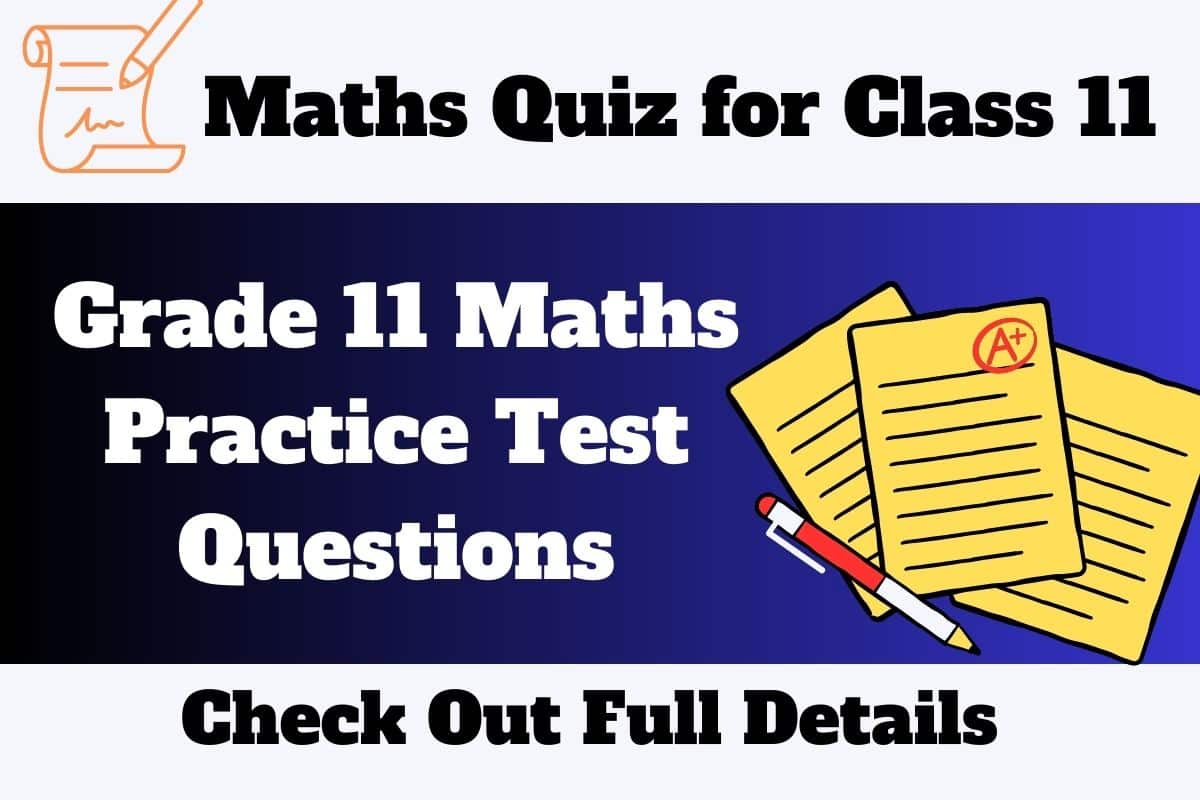WhatsApp Channel Download NowTelegram Group Join Now

# Maths Quiz for Class 11

Those candidates who are pursuing Class 11th and looking for Maths Quiz for Class 11 With Answers are at the correct page. Multiple Choice Questions (MCQs) of Grade 11th Maths Subject are given here, so that candidates can prepare well for their examinations.

Students can go through the given Practice Test Questions and match out their selected option with the answers given at the end of this page.

recruitmentresult.comMaths Quiz for Class 11 is prepared by keeping in mind that the questions given here will give an overview of all the chapters. Students can also download Maths Grade 11 Questions and Answers PDF from the below part of this page. So, let’s start out solving Maths Practice Test for Class 11.

## Maths Quiz for Class 11

Answers for these questions are available at the end.

Maths Quiz Questions with answers for Class 11/ Grade 11 Maths Questions and Answers are given here.

Question 1) The number of constant functions from a set containing m elements to a set containing n elements is

1. mn
2. m
3. n
4. m+n

Question 2) The function f:[0,2π]➝[-1,1] defined by f(x)=sin x is

1. one-to-one
2. on to
3. bijection
4. cannot be defined

Question 3) Let X={1,2,3,4}, Y={a,b,c,d} and f={f(1,a),(4,b),(2,c),(3,d),(2,d)}. Then f is

1. an one-to-one function
2. an onto function
3. a function which is not one-to-one
4. not a function

Question 4) Let f:R➝R be defined by f(x)=1-|x|. Then the range of f is

1. R
2. (1,∞)
3. (-1,∞)
4. (-∞,1]

Question 5) If |x+2| ≤ 9, then x belongs to

1. (−∞,−7)
2. [-11, 7]
3. (−∞,−7)∪(11,∞)
4. (-11, 7)

Question 6) The solution 5x-1<24 and 5x+1 > -24 is

1. (4,5)
2. (-5,-4)
3. (-5,5)
4. (-5,4)

Question 7) The value of loga b logb c logc a is

1. 2
2. 1
3. 3
4. 4

Question 8) cos10+cos20+cos30+: : :+cos1790=

1. 0
2. 1
3. -1
4. 89

Question 9) Which of the following is not true?

1. sinፀ=-3/4
2. cosፀ=-1
3. tanፀ=25
4. secፀ=1/4

Question 10) Let fk(x)=1/k[sinkx+coskx] where x∈R and k≥1. Then f4(x)-f6(x)=

1. 1/4
2. 1/12
3. 1/6
4. 1/3

Question 11) The sum of the digits at the 10th place of all numbers formed with the help of 2, 4, 5, 7 taken all at a time is

1. 432
2. 108
3. 36
4. 18

Question 12) The number of ways in which the following prize be given to a class of 30 boys first and second in mathematics, first and second in physics, first in chemistry and first in English is

1. 304× 292
2. 303× 293
3. 302× 294
4. 30×295

Question 13) The product of r consecutive positive integers is divisible by

1. r!
2. (r-1)!
3. (r+1)!
4. rr

Question 14) There are 10 points in a plane and 4 of them are collinear. The number of straight lines joining any two points is

1. 45
2. 40
3. 39
4. 38

Question 15) In 3 fingers, the number of ways four rings can be worn is ways.

1. 43-1
2. 34
3. 68
4. 64

Question 16) If a, 8, b are in AP, a, 4, b are in GP, and if a, x, b are in HP then x is

1. 2
2. 1
3. 4
4. 16

Question 17) The HM of two positive numbers whose AM and GM are 16,8 respectively is

1. 10
2. 6
3. 5
4. 4

Question 18) The remainder when 3815 is divided by 13 is

1. 12
2. 1
3. 11
4. 5

Question 19) If Sn denotes the sum of n terms of an AP whose common difference is d, the value of Sn-2Sn-1+Sn-2 is

1. 0
2. 2d
3. 4d
4. d2

Question 20) The equation of the locus of the point whose distance from y-axis is half the distance from origin is

1. x2+3y=0
2. x2-3y2=0
3. 3x2+y2=0
4. 3x2-y2=0

Want to Know? How to Prepare For Maths Exam

Question 21) Which of the following point lie on the locus of 3x2+3y2-8x-12y+17 = 0

1. (0,0)
2. (-2,3)
3. (1,2)
4. (0,-1)

Question 22) Straight line joining the points (2, 3) and (-1, 4) passes through the point (α,β) if

1. α+2=7
2. 3α+β=9
3. α+3β=11
4. 3α+β=11

Question 23) Equation of the straight line that forms an isosceles triangle with coordinate axes in the I-quadrant with perimeter 4 + 2√2 is

1. x+y+2=0
2. x+y-2=0
3. x+y−√2=0
4. x+y+√2=0

Question 24) The intercepts of the perpendicular bisector of the line segment joining (1, 2) and (3,4) with coordinate axes are

1. 5,-5
2. 5,5
3. 5,3
4. 5,-4

Also Check Out: List Of Important Maths Formulas

Question 25) If A and B are two matrices such that A + B and AB are both defined, then

1. A and B are two matrices not necessarily of same order
2. A and B are square matrices of same order
3. Number of columns of A is equal to the number of rows of B
4. A = B

### Maths Quiz for Class 11 with Answers:

 Ans 1) 3) Ans 10) 2) Ans 19) 1) Ans 2) 2) Ans 11) 2) Ans 20) 4) Ans 3) 4) Ans 12) 1) Ans 21) 3) Ans 4) 4) Ans 13) 1) Ans 22) 3) Ans 5) 2) Ans 14) 2) Ans 23) 2) Ans 6) 3) Ans 15) 2) Ans 24) 2) Ans 7) 2) Ans 16) 1) Ans 25) 2) Ans 8) 1) Ans 17) 4) Ans 9) 4) Ans 18) 2)